# PID Controllers in BESTune

The following PID controllers can be optimized by using the excellent PID auto-tuning software BESTune (see http://bestune.50megs.com for details). Theoretically, BESTune is able to optimize any PID controllers, as long as the PID equations implemented in them are known. In order to include more PID controllers in BESTune, I am asking you to give me more information about other well-known brands of industrial PID controllers (brand names, PID equations implemented, units of the three PID constants, etc). Your help will be very much appreciated.

Danyang Liu

BESTune.Com

Email: bestune@netzero.net

Telephone: +1-780-424-5074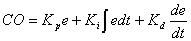where

 Kp: Proportional gain No unit Ki: Integral gain (1/second) Kd: Derivative gain (seconds)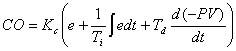where

 Kc: Proportional gain No unit Ti: Reset time (min/rep) Td: Rate time (min)

Allen Bradley PLC5 Independent PID - Using Integer Blocks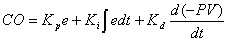where

 Kp: Proportional gain (0.01) Ki: Integral gain (0.001/ second) Kd: Derivative gain (seconds)

Allen Bradley PLC5 Independent PID - Using PD Blockswhere

 Kp: Proportional gain No unit Ki: Integral gain (1/ second) Kd: Derivative gain (seconds)

Allen Bradley PLC5 ISA PID - Using Integer Blockswhere

 Kc: Proportional gain (0.01) Ti: Reset time (0.01min/rep) Td: Rate time (0.01min)

Allen Bradley PLC5 ISA PID - Using PD Blockswhere

 Kc: Proportional gain No unit Ti: Reset time (min/rep) Td: Rate time (min)

Allen Bradley SLC5/02,SLC5/03 and SLC5/04 ISA PIDwhere

 Kc: Proportional gain (0.1) Ti: Reset time (0.1min/rep) Td: Rate time (0.01min)

Bailey Function Code FC19 with K=1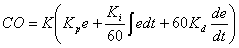where

 K: Gain multiplier No unit Kp: Proportional gain No unit Ki: Integral reset 1/min Kd: Derivative rate action Min

Bailey Function Code FC156 Independent Form with K=1where

 K: Gain multiplier No unit Kp: Proportional gain No unit Ki: Integral reset Resets/min Kd: Derivative rate action Min

Concept PID1 - PID Controller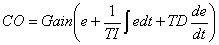where

 Gain: Proportional gain No unit TI: Reset time (milliseconds) TD: Derivative Action time (milliseconds)

Concept PID1P - PID Controller with parallel structure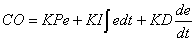where

 KP: Proportional gain No unit KI: Integral rate (1/milliseconds) KD: Differentiation rate (milliseconds)

Fischer & Porter DCU 3200 CON Ideal with KP = 1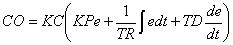If Kp = 1, the above equation reduces to:where

 KC: Gain constant No unit TR: Reset time (min/rep) TD: Derivative term (min)

Fischer & Porter DCU 3200 CON Parallel KP variable with KC=1If KC=1, the above equation reduces to: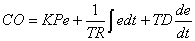where

 KP: Proportional gain No unit TR: Reset time (min/rep) TD: Derivative term (min)

GE Fanuc Series 90-30 and 90-70 Independent Form PID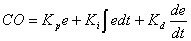where

 Kp: Proportional gain (0.01) Ki: Reset time (0.001rep/second) Kd: Derivative gain (0.01 seconds)

Hartmann & Braun Freelance 2000 PID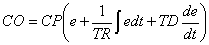where

 CP: Proportional correction value No unit TR: Reset time (milliseconds) TD: Rate time (milliseconds)

Honeywell TDC 3000 APM Non - Interactive PID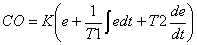where

 K: Gain No unit T1: Integral time constant (min/rep) T2: Derivative time constant (min)

### Modicon 984 PLC PID2 Equationwhere

 PB: Proportional band No unit K2: Integral mode gain constant (0.01min/rep) K3: Derivative mode gain constant (0.01min)

Siemens S7 PB41 CONT_C PID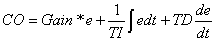where

 Gain: Proportional gain No unit TI: Reset time (seconds) TD: Derivative time (seconds)

### Yokogawa Field Control Station (FCS) PID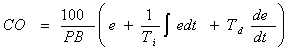where

 PB: Proportional band No unit Ti: Integral time (seconds) Td: Derivative time (seconds)